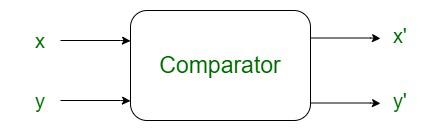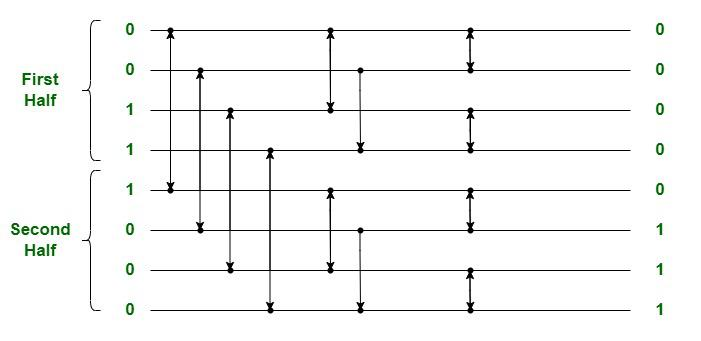GFG App
Open AppBrowser
Continue

# Bitonic Sorting Network using Parallel Computing

## What are sorting networks in parallel computing?

Sorting networks are comparison networks that always sort their inputs. They are also known as comparison networks. Comparison networks consist of wires and comparators. Wires are responsible to transmit value from one node to the other and a comparator is responsible to compare two inputs to produce 2 outputs.

A basic block diagram of a comparator is shown below:Comparator

In the above diagram:

• x and y are inputs
• x’ and y’ are outputs
• x’ = min(x, y)
• y’ = max(x, y)

In a sorting network, the output sequence is monotonically increasing. A Comparison network is like a procedure that specifies how comparisons occur.

Depth of a sorting network – It is the minimum number of comparators on a path from input wires to an output wire.

## Bitonic Sorting Network

It is a special type of sorting network. It consists of a bitonic sorter and a bitonic sequence. Let us see what is a bitonic sorter and bitonic sequence.

Bitonic Sorter – A bitonic sorter is composed of several stages each of which is called a half cleaner. Half cleaner is a comparison network of depth 1. Bitonic sorter is obtained by recursively combining half cleaners in which line i is compared with line (i + n/2) for all i in the range of [1, n/2].

Bitonic Sequence – Bitonic sequence is a sequence of bits i.e. 0 and 1s. It is a sequence that monotonically increases and then monotonically decreases or can be circularly shifted to become monotonically increasing and then monotonically decreasing.

## How bitonic sorting network works?

When a bitonic sequence of 0s and 1s is applied as an input to a half cleaner in a bitonic network, the half cleaner produces an output sequence in which smaller values are on the top half and larger values are on the bottom half and both halves are bitonic. Working of a bitonic sorting network can be seen with the help of an example.

Example:

Given a sequence of {0, 0, 1, 1, 1, 0, 0, 0} what will be the output when it will be passed through a bitonic sorting network?

Solution:Working of a bitonic sorting network

In the given example, the input is divided into two halves and the inputs of each half are compared in order and recursively until the input is completely sorted. The comparisons occur for log2 x where x is the number of inputs.

## Properties of the output of the bitonic sorting network:

• Both top and bottom half are clean.
• Every element in the top half is at least as small as every element in the bottom half.
• At least one half is clean (either all 0s or all 1s).
My Personal Notes arrow_drop_up